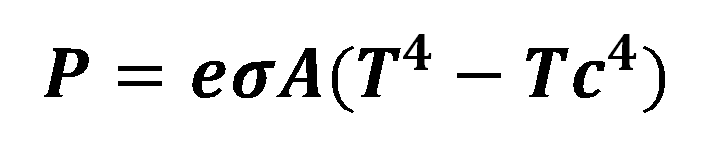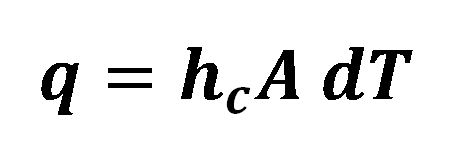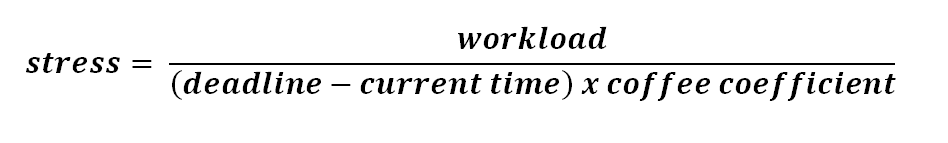# Everyday Formulas That All Engineering Students UseOK, cards on the table. With a such a broad brush we couldn't possibly cover all the key equations required for every engineering discipline in one article. Instead, we'll have a little fun and have a look at some formulas that all students will, likely, have needed to deal with on a day to day basis. They will range from thermodynamics to economics and will pertain to your daily lives rather than practical uses in your studies, or maybe you do?

Depending on your discipline we think we've covered the most basic ones that you'll all have "used" or "encountered" throughout your study days. Bear with us and let's have some fun with maths.

## Formula or equation

This might seem like a silly question but it's an interesting tangent to go off on briefly. These terms are often used interchangeably. Technically speaking an equation is any expression with an equals sign. Formulas, on the other hand, are intended to be a set of instructions to get a result and may include an equals sign, or not. Formulas are intended to show the relationship between the variables within them. Equations will, on the whole, include more known variables than formulas. The next logical question is should it be formulas or formulae? That's a question for another day.

With that in mind, the following should be thought of as formulas in the form of an equation.

Let's take a look at the most important equations that have the greatest effects on your studies, well maybe not.

## Your ever cooling caffeinated beverage

Coffee and tea are an essential study tool during College, University and frankly, the rest of your life. Here are the key formulas that will constantly require you to time your "sip" breaks and refill. As we know heat is transferred by conduction, convection, radiation and evaporation for fluids, but we'll ignore the latter for brevity.[Image Source: Pixabay]

## Heat Loss by Thermal Conduction

Formula:Q = Amount of heat transferred

t = Time

k = Thermal conductivity of the material

A = Area

T1 = Temperature on one side the material

T2 = Temperature on the other side

d = Thickness of material

## Heat Loss by Thermal Radiation - Stefan-Boltzmann Law

Formula:e = Emissivity (1 for an ideal radiator)

o = Stefan's constant (5.6703 x 10-8 watt/m2K4)

Tc = Temperature of surroundings

## Thermal Convection - Newton's Law of Cooling

Formula:q = heat transferred per unit time (W, Btu/hr)

A = heat transfer area of the surface (m2, ft2)

hc = convective heat transfer coefficient of the process (W/(m2K) or W/(m2oC), Btu/(ft2 h oF))

dT = temperature difference between the surface and the bulk fluid (K or oC, F)

Ok time for some more serious ones. A quick poll of our team shows the following formulas are actually used far more often than you might initially expect.

## Stress[Image Source: Pixabay]

No, not that kind, well maybe from the perspective of your chair or elbows. Stress is a measure of the internal force an object is experiencing per unit cross-sectional area. This formula for calculating stress is the same as the formula for calculating pressure.

Formula:Where:

Stress is expressed in Newtons per square meter or Pascals

Force is expressed in Newtons

Area is expressed in cross-sectional area.

Any stress applied to a material is measured in force per unit area applied to the material. All materials will have a maximum stress they can withstand before breaking. This simple yet fundamental equation has applications in most, if not all fields of engineering and most of probably use this unwittingly every single day.

For the other kind we could perhaps calculate it as follows:## Pythagoras's theorem

If I had a penny for every time I've needed to use this formula I'd be, well, a bit richer. A fantastically simple one to remember. It's often invoked when we need to calculate areas and volumes of, well anything with cheeky triangular elements. Frankly, Pythagoras's theorem is a very underrated formula and deserves some respect, you hear!

Formula:- a2 = b2 + c2 (plus rearrangements for known lengths)

c = length of the hypotenuse

a and b = length of either of the other two sides[Image source: Pixabay]

## Compound Interest

Look, let's face it. Unless your entire university experience was bankrolled by your parents or a generous patron, most students effectively live off debt. Whether it be a student loan or the very embodiment of evil, credit cards. Until such time that basic financial education is an integral part of our education, most students use this formula throughout their University days and beyond. Compound interest is awesome for investors but it's a double-edged sword. Interest on any loan will abide by the following formula:

Formula for compound interest plus principle:  A = P (1 + r/n) (nt)

For compound interest only: P (1 + r/n) (nt) - P

A = the future value of the investment/loan, including interest
P = the principal investment amount (the initial deposit or loan amount)
r = the annual interest rate (decimal)
n = the number of times that interest is compounded per year
t = the number of years the money is invested or borrowed for

Just goes to show the importance of clearing those higher rate loans as soon as you can. Debts can, and will, quickly mount up. The higher the rate the quicker it will double magically before your very eyes. This might just be the most important formula you learn today, you're welcome.

Clearly, this list is a bit of fun. We have included some "series" formulas which will actually be of use to some of you. With such a large subject as this, it would be a good exercise to ask for your suggestions. Which formulas do you use on a daily basis? If you could talk to your younger self which ones would you drum into them? Let's start a conversation.